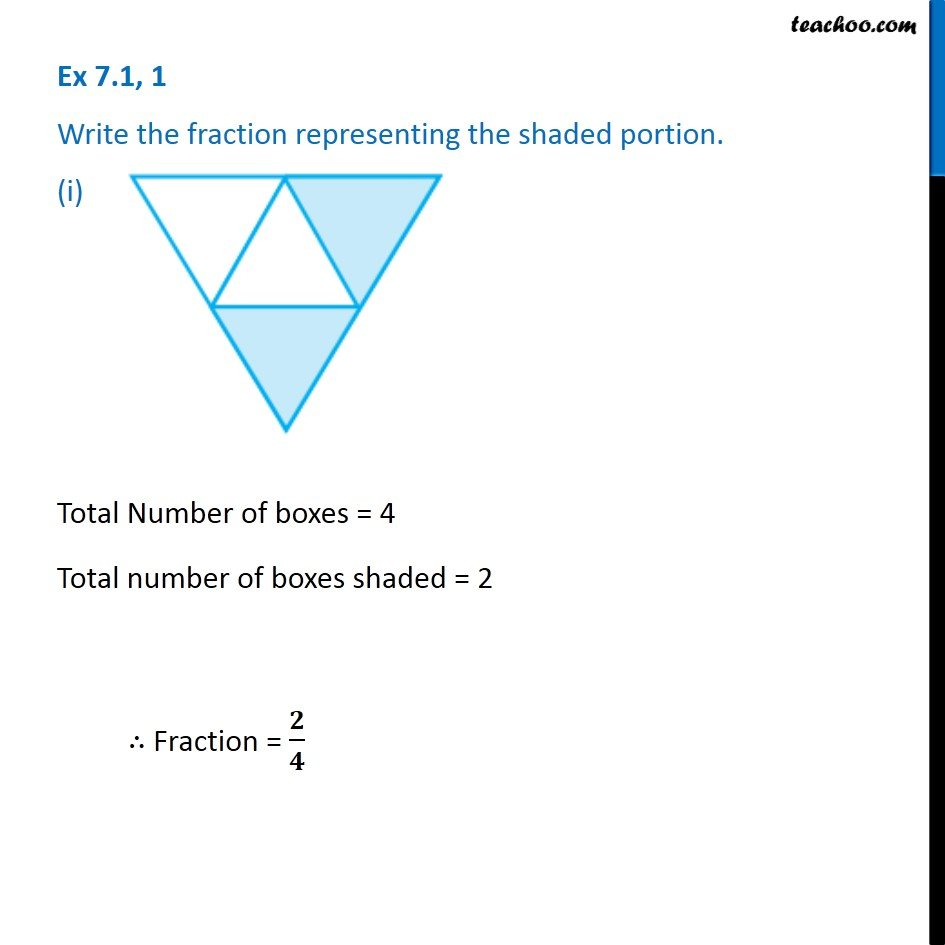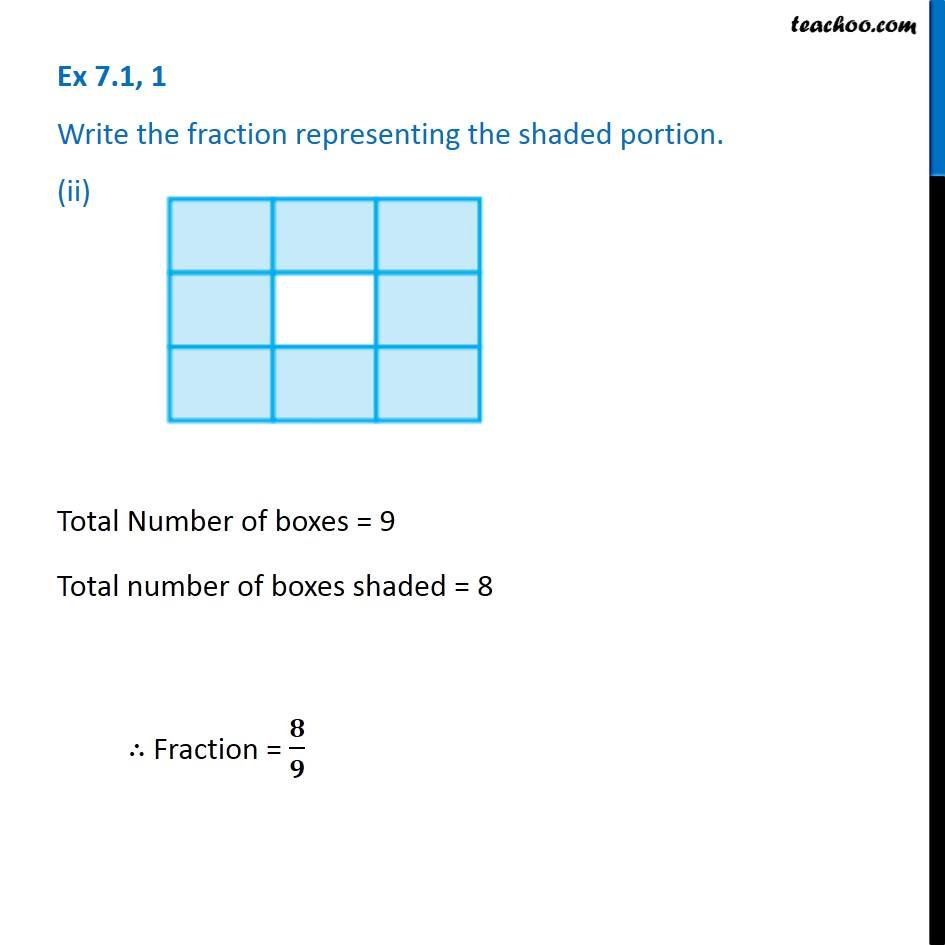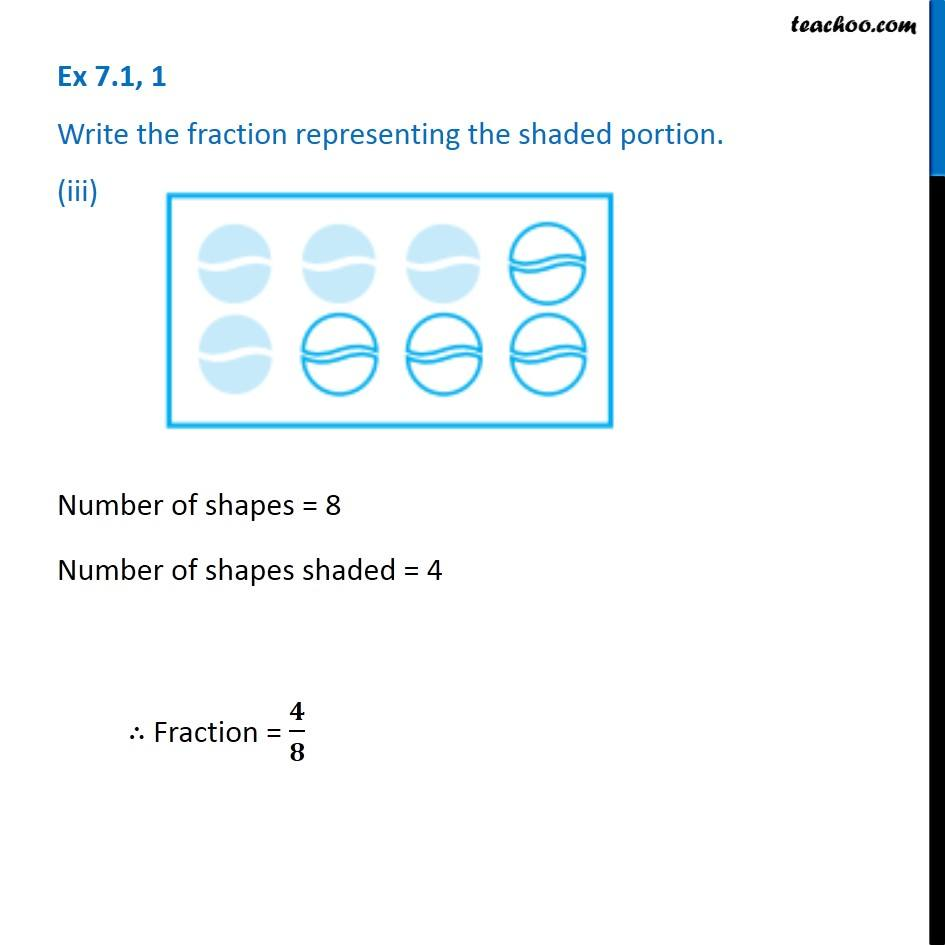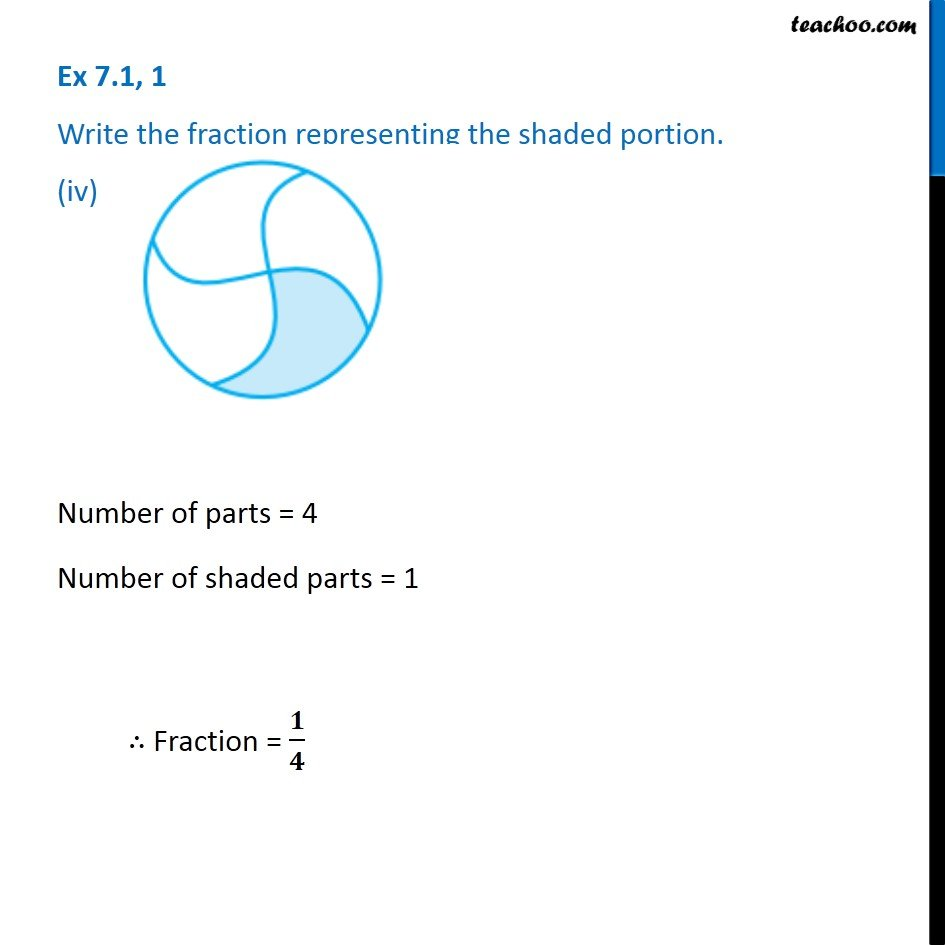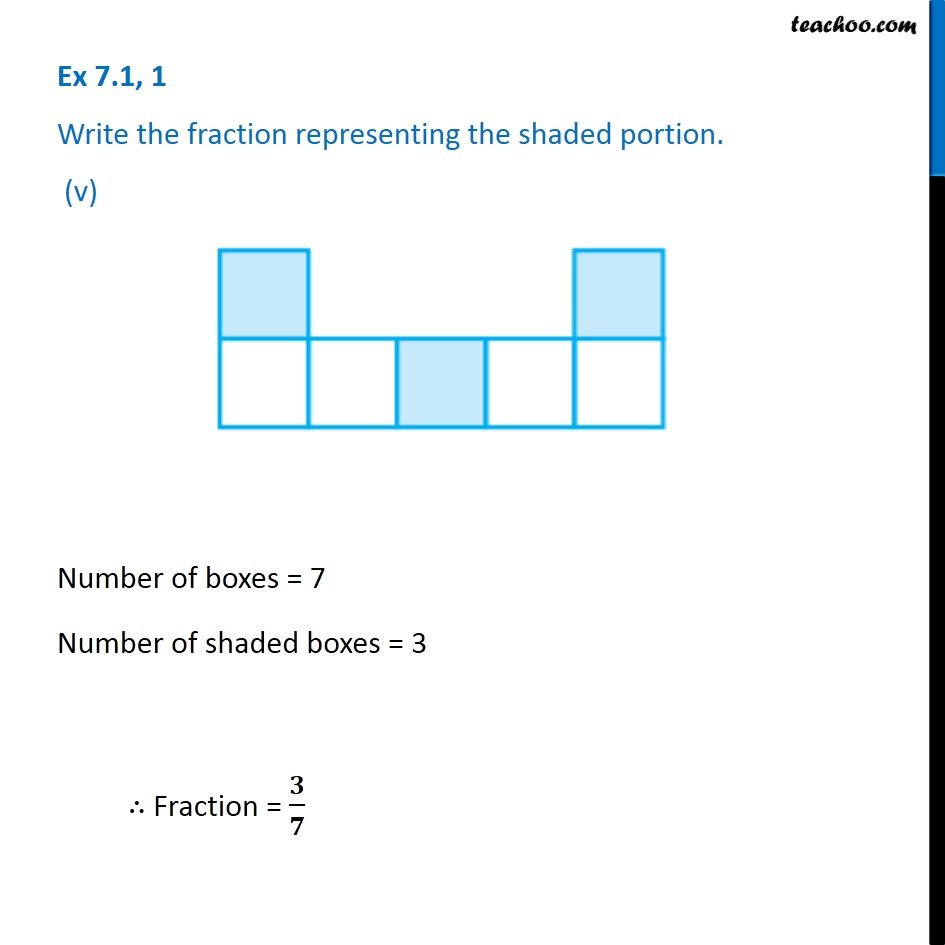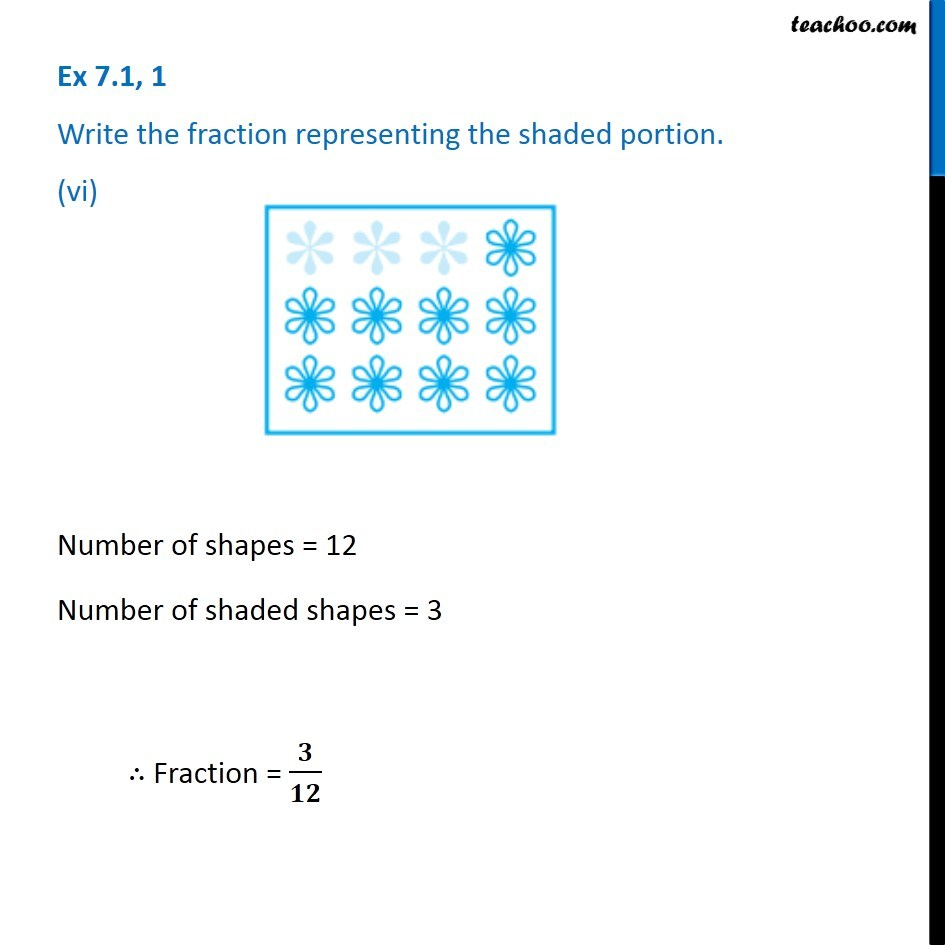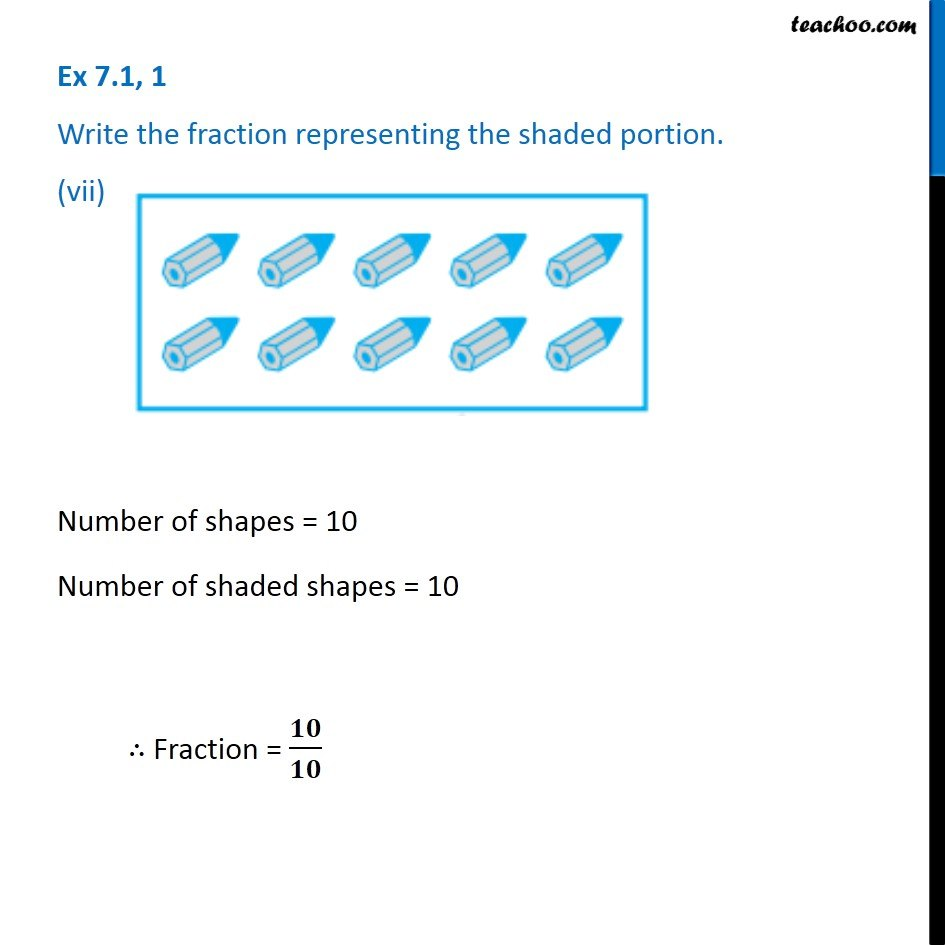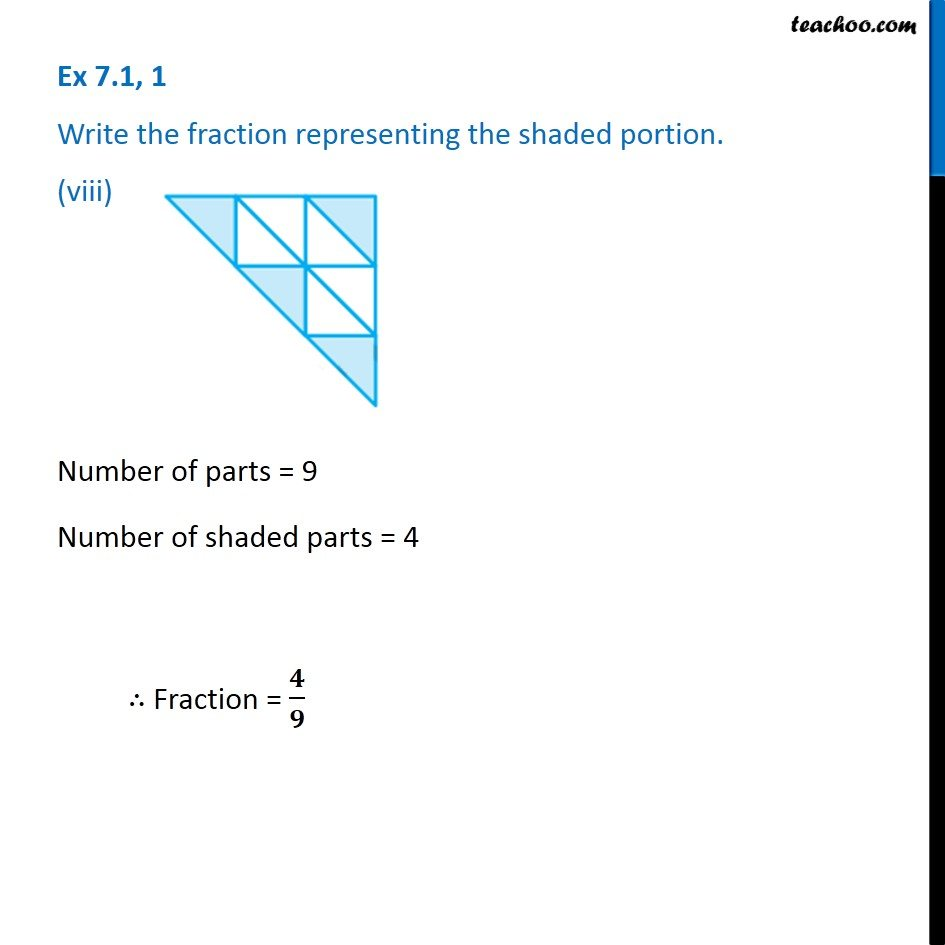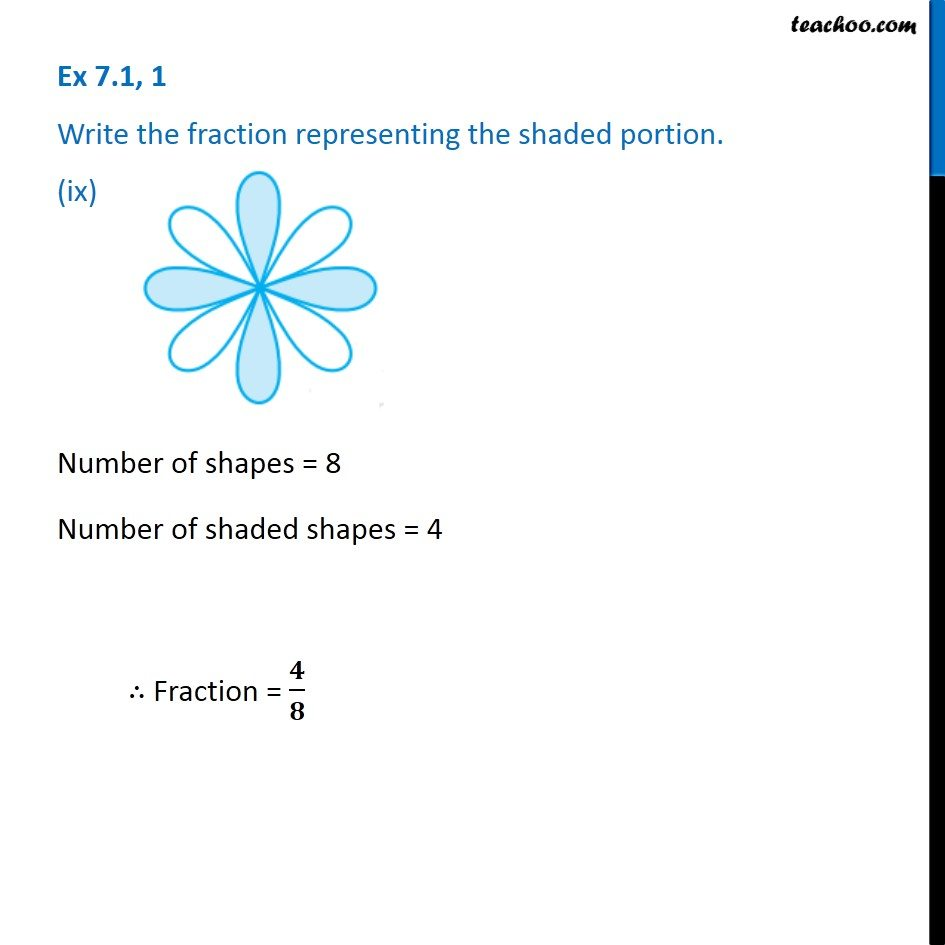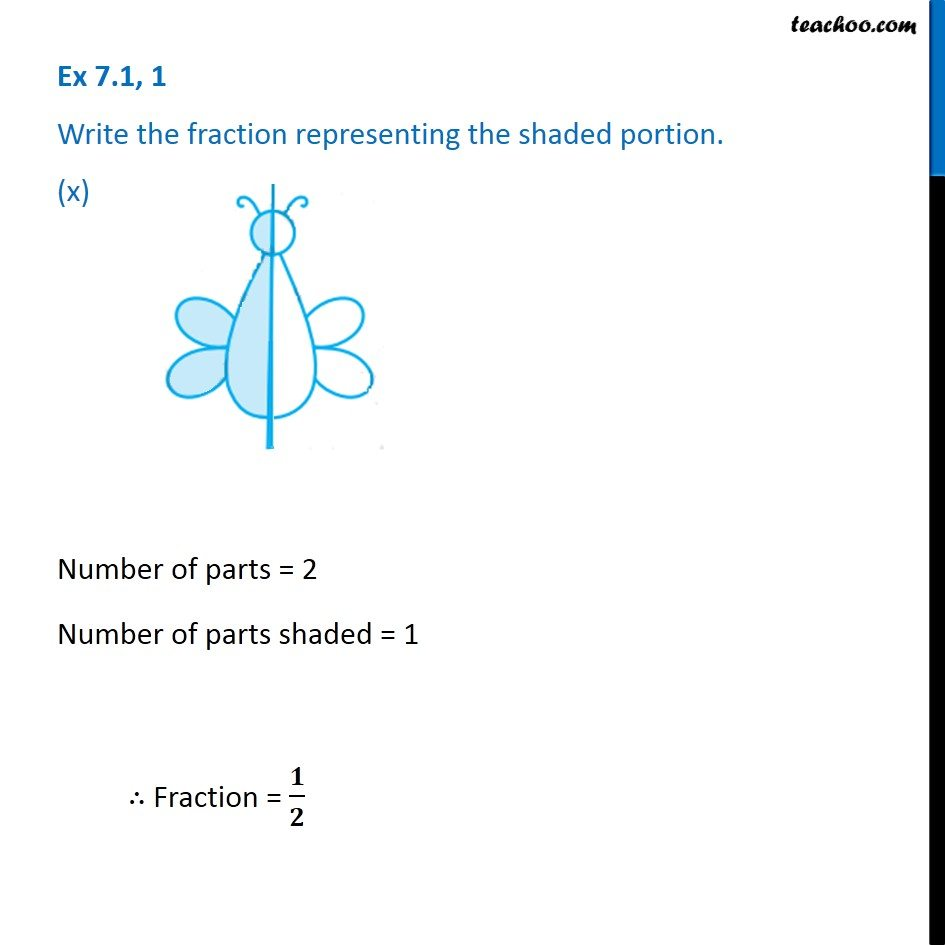1. Chapter 7 Class 6 Fractions
2. Serial order wise
3. Ex 7.1

Transcript

Ex 7.1, 1 Write the fraction representing the shaded portion. (i) Total Number of boxes = 4 Total number of boxes shaded = 2 ∴ Fraction = 𝟐/𝟒 Ex 7.1, 1 Write the fraction representing the shaded portion. (ii) Total Number of boxes = 9 Total number of boxes shaded = 8 ∴ Fraction = 𝟖/𝟗 Ex 7.1, 1 Write the fraction representing the shaded portion. (iii) Number of shapes = 8 Number of shapes shaded = 4 ∴ Fraction = 𝟒/𝟖 Ex 7.1, 1 Write the fraction representing the shaded portion. (iv) Number of parts = 4 Number of shaded parts = 1 ∴ Fraction = 𝟏/𝟒 Ex 7.1, 1 Write the fraction representing the shaded portion. (v) Number of boxes = 7 Number of shaded boxes = 3 ∴ Fraction = 𝟑/𝟕 Ex 7.1, 1 Write the fraction representing the shaded portion. (vi) Number of shapes = 12 Number of shaded shapes = 3 ∴ Fraction = 𝟑/𝟏𝟐 Ex 7.1, 1 Write the fraction representing the shaded portion. (vii) Number of shapes = 10 Number of shaded shapes = 10 ∴ Fraction = 𝟏𝟎/𝟏𝟎 Ex 7.1, 1 Write the fraction representing the shaded portion. (viii) Number of parts = 9 Number of shaded parts = 4 ∴ Fraction = 𝟒/𝟗 Ex 7.1, 1 Write the fraction representing the shaded portion. (ix) Number of shapes = 8 Number of shaded shapes = 4 ∴ Fraction = 𝟒/𝟖 Ex 7.1, 1 Write the fraction representing the shaded portion. (x) Number of parts = 2 Number of parts shaded = 1 ∴ Fraction = 𝟏/𝟐

Ex 7.1

Chapter 7 Class 6 Fractions
Serial order wise

About the AuthorDavneet Singh
Davneet Singh is a graduate from Indian Institute of Technology, Kanpur. He has been teaching from the past 9 years. He provides courses for Maths and Science at Teachoo.